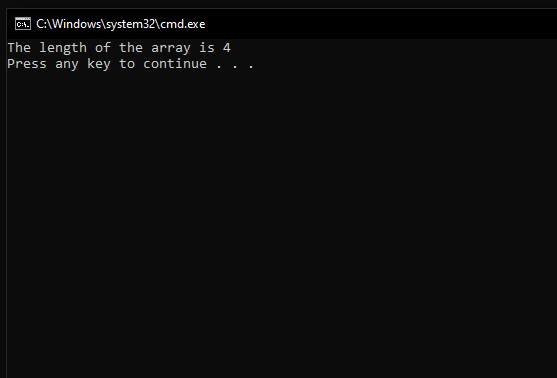Batch Script – Length of an Array

• Last Updated : 28 Nov, 2021

The length of the array is the number of elements in the array. The index of the array starts from “0” to “N-1” where N is a number of elements.

For example

arr=1
arr=2
arr=3

Here we can see that the index starts from 0 and ends with 2 . So, we know that the index of the element goes from 0 to N-1. Now, N-1=2, and hence the value of N becomes 3 i.e. N=3, where N is number of elements in the array.

How to find the length of the array using batch script

In the batch script, there is no function to find the length of the array direct so we have to iterate the elements of the array.

First, open the notepad and write the below command.

@echo off
:: Here an array is defined
set array=1
set array=4
set array=9
set array=10

:: Here we initializing an variable named len to calculate length of array
set len=0

:: To iterate the element of array
:Loop

:: It will check if the element is defined or not
if defined array[%len%] (
set /a len+=1
GOTO :Loop
)
echo The length of the array is %len%
pause

Save the above file with “.bat” extension and run the file.

Output :

The length of the array is 4Explanation :

• First, we create an array to calculate the length.
• After this, we have to initialize a variable to calculate the length of the array. Above we initialize len=0
• Now we have to iterate the elements of the array to calculate the length of the array.
• To check the element is exists or not in the array we have to put an if statement as shown in the above code.
• In case if statement is true then there is an increment in len.
• In case if statement is false then it will exit from the loop and return the length of the array.
My Personal Notes arrow_drop_up